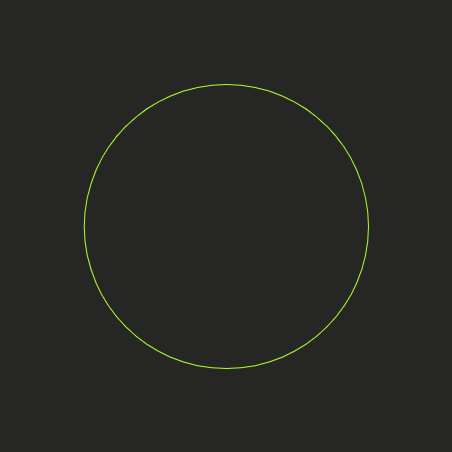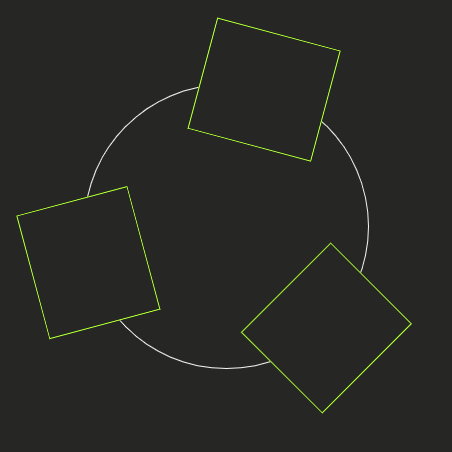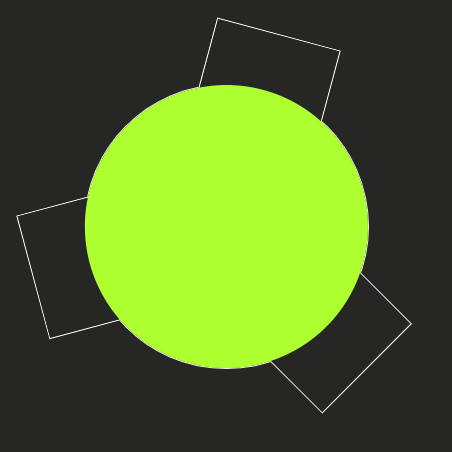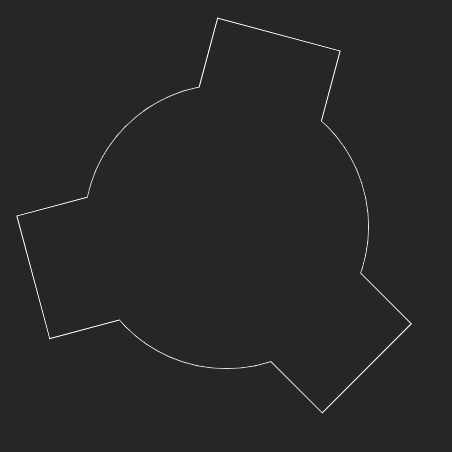A gear or cogwheel is a rotating machine part having cut teeth or, in the case of a cogwheel, inserted teeth (called cogs), which mesh with another toothed part to transmit torque.

In this article, I will show how to build an interactive gear-shaped object.

To catch the idea, let's consider the gear as a circularly placed set of teeth.

Each tooth has its characteristics, such as shape and height.

Having the above data in mind, let's build such an object.

# HTML

The static part of the layout is simple. We will only define the container which we will set up and fill with objects.

``````<!DOCTYPE html>
<html lang="en">
<meta charset="UTF-8" />
<meta name="viewport" content="width=device-width, initial-scale=1.0" />
<meta http-equiv="X-UA-Compatible" content="ie=edge" />
<title>Gear</title>
<body>
<div id="container"></div>
</body>
</html>
``````

The dynamic part will contain tooth:

``````<div
class="tooth"
style="height: 5vmin; width: 14.5vmin; transform: rotateZ(315deg) translateX(15vmin);"
></div>
``````

And a cover of the central part:

``````<div class="cover"></div>
``````

Part of teeth parameters is calculated by JavaScript, while the rest of the settings are defined by CSS.

# CSS

First of all, we will define basic settings, to have an ability to adjust our object by altering data in a single place.

``````:root {
--smokey: # f5f5f5;
--darky: # 262625;
--thickness: 0.1vmin;
--half: 50%;
--border: var(--thickness) solid var(--smokey);
}
``````

## Container

The container not only contains teeth but also acts as an outer fringe of the gear's main body.

``````# container {
position: relative;
display: flex;
border: var(--border);
justify-content: center;
align-items: center;
}
``````

To form a circular shape of the container, we will set the border-radius to 50%. Also, we will apply the border rule.

## Cover

The cover helps us to create a single gear outline. To get the idea, let's take a look at the layout layer by layer.

The first layer is a container with the border.The next layer contains a set of teeth. The inner half of each tooth is placed inside the container. Thus, creating a single outline.The last layer contains the cover element, which hides the inner part of the teeth.So, by placing objects in corresponding layers, and by setting the correct background-color, we are creating a single outline via hiding unnecessary parts.Since the gear is rebuilt anew after any of the parameters alterations, it is worth to mention that the cover element requires to be set of the proper z-index value.

Let's wrap it up:

``````# container .cover {
position: relative;
display: flex;
justify-content: center;
align-items: center;
width: 100%;
height: 100%;
background: var(--darky);
z-index: 1;
}
``````

It is assumed that gear must be mounted on a shaft.

So, next, we will add the landing hole.

To keep the layout simple let's use a pseudo-element before of the cover element:

``````# container .cover::before {
width: var(--half);
height: var(--half);
content: "";
border: var(--border);
}
``````

To center our landing hole we use the flex properties of the cover element.

## Tooth

Last but not least element of our shape is a tooth.

While most of the set up is happening in the JavaScript part, there still are some CSS rules.

First of all, the tooth element has an absolute position. Secondly, we leverage the box-sizing CSS rule to not to break the layout.

``````# container .tooth {
position: absolute;
box-sizing: border-box;
}
``````

Just for fun, I've added three types of shapes of teeth: square, circle, and triangle.

Each type of shape is built via the before pseudo-element.

### Square

This is the default type, so it doesn't have a separate class name.

This is a bordered square with an absolute position:

``````# container .tooth::before {
position: absolute;
width: 100%;
height: 100%;
border: var(--border);
content: "";
background: var(--darky);
}
``````

### Circle

In the case of the circle, we will apply a border-radius trick:

``````# container .tooth.circle::before {
}
``````

### Triangle

To turn the square into the triangle let's just rotate it 45 degrees:

``````# container .tooth.triangle::before {
transform: rotateZ(45deg);
}
``````

# JavaScript

The core variables are stored globally. They are defining all of the parameters of our object: DOM reference to the container, the radius of the gear, number of teeth, height and shape of a tooth, outline thickness, and the angle of the gear rotation.

The API consists of the set of functions. Some of them are very basic and aimed to handle user input: setTeeth, setHeight, setShape, and setAngle. Here is an example of such function:

``````/**
* set modifier for tooth height
* @param {number} value tooth height modifier
*/
function setHeight(value) {
height = value;
update();
}
``````

It is worth to mention the setThickness function because it alters the value of the CSS variable --thickness:

``````/**
* set thickness
* @param {number} value thickness value
*/
function setThickness(value) {
document.documentElement.style.setProperty(
"--thickness",
`
\${value / 10}vmin
`
);
}
``````

The heavy-duty function that builds the gear has name update.

We will break it into steps to understand what happens.

Before actions, we will calculate the base settings.

Firstly, we need to know the dimensions of the container. Next, we will find out the values of the teeth' basic parameters.

``````// calculate the container dimensions
const size = `
`;
// calculate the angle between teeth
const step = 360 / teeth;
// calculate the base dimension of the tooth
const side = (2 * Math.PI * radius) / (teeth * (Math.PI / 2));
// calculate the tooth displacement
const displacement = radius * 1.5;
// calculate the height multiplier
const multiplier = (height - 1) / 10;
``````

Next, let's set up the container:

``````// setup container
container.style.width = size;
container.style.height = size;
container.style.margin = `
`;
container.style.transform = `
rotate(\${angle}deg)
`;
container.innerHTML = null;
``````

Now we will draw teeth:

• create the element.
• apply proper class names.
• set the width and height following the current shape.
• rotate the tooth and place it on the rim.
• add tooth to the container.
``````// create tooth
const tooth = document.createElement("div");
tooth.className = `
tooth \${shape}
`;
// set size for the triangle-shaped tooth
if (shape === "triangle") {
const length = `
\${(side / 2) * multiplier}vmin
`;
tooth.style.height = length;
tooth.style.width = length;
} else {
// set size for the square and circle-shaped teeth
tooth.style.height = `
\${side}vmin
`;
tooth.style.width = `
\${side * multiplier}vmin
`;
}
// place the tooth
tooth.style.transform = `
rotateZ(\${i *
step}deg) translateX(\${displacement}vmin)
`;
// append tooth to the container
container.appendChild(tooth);
``````

When we set up the width and height of a tooth, we rely on the side constant. The point here is to draw teeth in strict proportion to their count to avoid overlay. So, the more teeth you have, the smaller they are. Another point is that this calculation is also lead to the proportional reduction of the tooth height to keep it's looking more balanced.

Finally, add the cover element:

``````// restore cover
const cover = document.createElement("div");
cover.className = "cover";
container.appendChild(cover);
``````

Let's wrap it up:

``````/**
* update the gear
*/
function update() {
if (container) {
// calculate the container dimensions
const size = `
`;
// calculate the angle between teeth
const step = 360 / teeth;
// calculate the base dimension of the tooth
const side = (2 * Math.PI * radius) / (teeth * (Math.PI / 2));
// calculate the tooth displacement
const displacement = radius * 1.5;
// calculate the height multiplier
const multiplier = (height - 1) / 10;
// setup container
container.style.width = size;
container.style.height = size;
container.style.margin = `
`;
container.style.transform = `
rotate(\${angle}deg)
`;
container.innerHTML = null;
// draw teeth
for (var i = 0; i < teeth; i++) {
// create tooth
const tooth = document.createElement("div");
tooth.className = `
tooth \${shape}
`;
// set size for the triangle-shaped tooth
if (shape === "triangle") {
const length = `
\${(side / 2) * multiplier}vmin
`;
tooth.style.height = length;
tooth.style.width = length;
} else {
// set size for the square and circle-shaped teeth
tooth.style.height = `
\${side}vmin
`;
tooth.style.width = `
\${side * multiplier}vmin
`;
}
// place the tooth
tooth.style.transform = `
rotateZ(\${i *
step}deg) translateX(\${displacement}vmin)
`;
// append tooth to the container
container.appendChild(tooth);
}
// restore cover
const cover = document.createElement("div");
cover.className = "cover";
container.appendChild(cover);
}
}
``````

# Conclusion

Now you know how to build a gear-shaped object.

Though I did not cover the controls in this article, you can use an API to dynamically modify the number of teeth, the rotation angle of the object, set the height of the tooth, choose from three shapes of the tooth, and set up the thickness of the outline.

This post is also available on DEV.